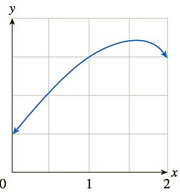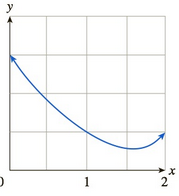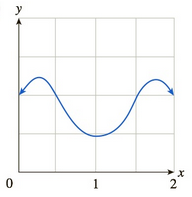Chapter 10.4, Problem 63EFinite Mathematics and Applied Cal...

7th Edition
Stefan Waner + 1 other
ISBN: 9781337274203

Solutions

Chapter
SectionFinite Mathematics and Applied Cal...

7th Edition
Stefan Waner + 1 other
ISBN: 9781337274203
Textbook Problem

A certain function f has the property that its average rate of change over the interval [ 1 , 1 + h ] (for positive h) increases as h decreases. Which of the following graphs could be the graph of f?(A)(B)(C)To determine

The correct graph of function f which has the property that the average rate of change over the interval [1,1+h] (for positive h) increases as h decreases out of the given options.

Explanation

Given information:

The options are:

A)

B)

C)

When function is increasing or rising the slope of the function is positive and greatest where the curve is more slanted whereas when the function is decreasing or falling its slope is negative and it has the least value where the curve is the steepest.

It is given that function has the property that the average rate of change over the interval [1,1+h] (for positive h) increases as h decreases.

The average rate of change of f is defined by f(1+h)f(1)h.

As the value of h decreases, the secant line for f(1+h) and f(1) become steeper and increasing

Still sussing out bartleby?

Check out a sample textbook solution.

See a sample solution

The Solution to Your Study Problems

Bartleby provides explanations to thousands of textbook problems written by our experts, many with advanced degrees!

Get Started